Courses

# Solid State & Surface Chemistry (22-11-2018)

## 30 Questions MCQ Test AAKSC Chemistry Mock test Series | Solid State & Surface Chemistry (22-11-2018)

Description
This mock test of Solid State & Surface Chemistry (22-11-2018) for Class 12 helps you for every Class 12 entrance exam. This contains 30 Multiple Choice Questions for Class 12 Solid State & Surface Chemistry (22-11-2018) (mcq) to study with solutions a complete question bank. The solved questions answers in this Solid State & Surface Chemistry (22-11-2018) quiz give you a good mix of easy questions and tough questions. Class 12 students definitely take this Solid State & Surface Chemistry (22-11-2018) exercise for a better result in the exam. You can find other Solid State & Surface Chemistry (22-11-2018) extra questions, long questions & short questions for Class 12 on EduRev as well by searching above.
QUESTION: 1

Solution:
QUESTION: 2

### Which one of the following methods is commonly used for destruction of colloid?

Solution:

Addition of electrolyte brings in coagulation of sol.

QUESTION: 3

### Select the correct statements : (i) greater is the valency of effective ion in electrolyte more will be coagulation power of electrolyte for a given sol. (ii) greater is the valency of effective ion in electrolyte lesser will be its coagulation value for a given sol. (iii) peptisation is a characteristic property of colloidal solution. (iv) micelle formation occurs only above Kraft’s temperature as well as above critical micelle concentration. (v) lower is the gold number more is protecting power of colloid.

Solution:

Peptisation is a method to prepare sol.

QUESTION: 4

How many ‘nearest’ and ‘next nearest’ neighbours respectively potassium have in bcc lattice?

Solution:

Nearest neighbours are corners & next nearest are body centres of adjacent unit cell, in bcc lattice.

QUESTION: 5

A binary solid (A+ B-) has a zinc blends structure with B- ions constiuting the lattice and A+ ions occupying 25% tetrahedral holes. The formula of solids is:

Solution:

No. of B- ions in unit cell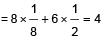Now A+ ions occupies 25% of tetrahedral holes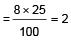Thus, ratio of B- to  AA+ is 2 : 1 or formula is AB2

QUESTION: 6

Which of the following statement is not correct?

Solution:

No. of carbon atoms in unit cell of diamond is 8, as it follow ZnS type

QUESTION: 7

If NaCI is doped with 10-4 mole % of SrCI2, the concentration of cation vacancies will be :

Solution:

Dropping of SrCI2 to NaCI brings in replacement of two Na+ by each Sr2+ ion, but Sr2+ occupies one lattice point.
This produces one cation vacancy.
No. of cation vacancies = 10-4 mol%
100 mole of NaCI will have cationic vacancy = 10-4
∴ 1 mole of NaCI will have cationic vacancy = 10-4
∴ 100 = 10-6
∴ No. of cationic vacancies
= 10-6 x 6.02 x 1023 = 6.02 x 1017

QUESTION: 8

AB crystallises in a bcc lattice with edge length ‘a’ equal to 387 pm. The distance between two oppositely charged ions in the lattice is:

Solution:

For a bcc lattice,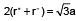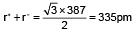QUESTION: 9

How many unit cells are present in a cube shaped ideal crystal of NaCI of mass 1.00 g?

[Atomic masses Na = 23, CI = 35.5]

Solution:

Mass of one unit cell = V x d (V is volume, d is density)
= a3 x d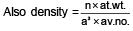∴ Mass of one unit cell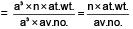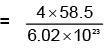(n = 4 for cubic shape)

= 38.87 x 10-23g

∴  No. of unit cell is 1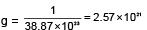QUESTION: 10

In a compound, atoms of element Y from ccp lattice and those of element X occupy 2/3rd of tetrahedral voids. The formula of  the compound will be:

Solution:

In a ccp array number of tetrahedral voids is twice the number of atoms.

QUESTION: 11

Copper crystallises in fcc with a unit cell length of 361 pm. What is the radius of copper atom?

Solution:

For fcc n = 4

and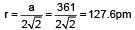QUESTION: 12

A metallic element crystallises into lattice containing a sequence of layers of ABABABAB......Any packing of spheres leaves out avpoid in the lattice. The empty space in percentage by volume on thid lattice is:

Solution:

ABABAB...packing has empty space of 48% in sc, 32% in bcc, 26% in hcp and ccp.

QUESTION: 13

Which statement is wrong?

Solution:

Hydrogenation of o ils requires Ni as catalyst

QUESTION: 14

Negative catalyst or inhibitor is one :

Solution:

The definition of negative catalyst.

QUESTION: 15

Which explains the effect of a catalyst on the rate of a reversible reaction?

Solution:

Follow th eories of catalysis.

QUESTION: 16

The unit cell length for LiCI (just like NaCI structure) is 5.14 , Assuming anion-anion contact, the ionic radius chloride ion is:

Solution:

The distance between Li+ and CI- ion can be derived as half of the edge length of cube.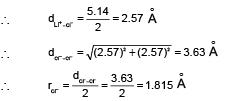QUESTION: 17

A solid XY has NaCI structure. If radius of X+ is 100 pm. What is the radius of Y- ion ?

Solution: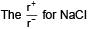= 0.414 to 0.732 (due to fcc structure)

QUESTION: 18

The ionic radii of Rb+ and I- are 1.46 and 2.16 Å. The most probable type of structure exhibited by it is:

Solution: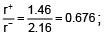Thus, it is fcc structure or NaCI type structure.

QUESTION: 19

Potassium has a bcc structure with nearest neighbour distance 4.52 Å . Its atomic weight is 39, its density will be :

Solution: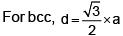or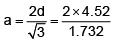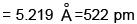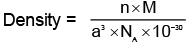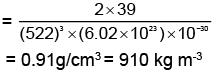QUESTION: 20

Which is not true in case of catalyst?

Solution:

Catalyst d oes not alter the equilibrium constant.

QUESTION: 21

Addition of FeCI3 to K4[Fe(CN)6] in dilute and cold solution gives:

Solution:

Fe4[Fe(CN)6]3 is Prussian blue sol of + ve charge

QUESTION: 22

Which is not a property of hydrophilic sols?

Solution:

Viscosity of hydrophilic sol is much higher than dispersion medium. Surface tension being muc h lesser than dispersion medium (water).

QUESTION: 23

Isoelectric point refers to the, concentration at which the colloidal particles:

Solution:

The sol particles at isoelectric point do not show electrophoresis.

QUESTION: 24

Bleeding is stopped by the application of ferric chloride. This is because:

Solution:

Fe3+ ion coagulates -ve sol particles of blood and seals the cut.

QUESTION: 25

Tanning of leather is:

Solution:

It is definition of tanning of leather.

QUESTION: 26

Which of the following is not a surfactant ?

Solution:

Rest all have hydrophillic head & hydrophobic tails.

QUESTION: 27

Which reaction gives colloidal solution?

Solution:

On passing H2S through a cold solution of an oxidant, colloidal sulphur is formed.

QUESTION: 28

How many tetrahedral holes are occupied in diamond?

Solution:

Diamond has ZnS structure so, 50% of tetrahedral voids are occupied with CN 4.

QUESTION: 29

Which of the following statements is not correct?

Solution:

In bcc structure CN is eight.

QUESTION: 30

In antifluorite structure, the negative ions:

Solution:

In antifluorite crystal (Na2O), the anions are arranged in cubic close packing while the cations occupy all the tetrahedral voids.